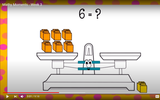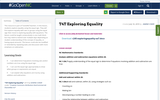Updating search results...

# 3 Results

View
Selected filters:
• SignUnrestricted Use
CC BY
Rating
0.0 stars
Overview:

This video lesson examines the meaning of the equal sign as we work to solve unknowns in multiplication and division equations.

Subject:
Mathematics
Material Type:
Activity/Lab
Interactive
Lesson
Simulation
Author:
Dawne Coker
06/26/2020Conditional Remix & Share Permitted
CC BY-NC-SA
Rating
0.0 stars
Overview:

This resource is part of Tools4NCTeachers.

In this lesson, students explore equality using the equal sign. They will first explore equality with sets or groups using the equal sign then move to exploring equality with equations. The lesson could be taught consecutively in one math block but may need to extend over multiple days depending on student understanding of the concept of equality, or having equal amounts. The lesson could also be modified or extended by repeating tasks and discussion with more emphasis on subtraction.

Subject:
Mathematics
Material Type:
Lesson
Lesson Plan
Author:
DAWNE COKER
11/06/2019Unrestricted Use
CC BY
Rating
4.0 stars
Overview:

This resource is from Tools4NCTeachers.In this lesson, students work with different quantities to determine if they have the same value / equal.  The information is presented in a context, not simply in an equation format, so students will need to apply their understanding of problem solving and resulting equations along with computation in order to determine if the amounts are equal.  Students will explain and defend their answers.  This file includes lesson, student recording sheets, and printable exit tickets.  Remix this lesson to include extension ideas or student work samples.

Subject:
Mathematics
Material Type:
Lesson
Lesson Plan
Author:
DAWNE COKER